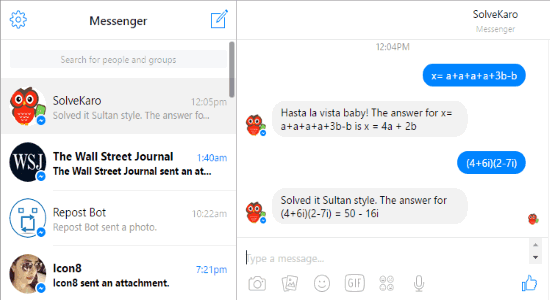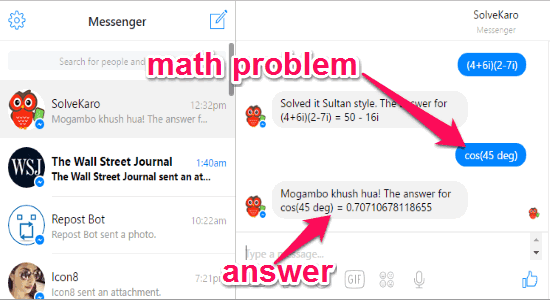Editor Ratings:
User Ratings:
[Total: 2 Average: 4]

SolveKaro is a free Facebook Messenger chatbot to solve math problems on Facebook Messenger. You can do operations like addition, division, subtraction, multiplication, linear equation with basic operators, exponent, factorial, basic trigonometric functions, quadratic & cubic equations, and more. Using this chatbot, you can easily perform the above mathematical calculations by simply typing the math problems on Facebook Messenger. However, there are various calculations which the chatbot cannot perform like natural language problems, linear equations with more than one variable, equations containing logs, equations with trigonometric functions, etc.

To calculate the supported mathematical operations, all you need to do is integrate this chatbot with your Facebook Messenger and then type math questions while chatting with the SolveKaro bot. No more using heavyweight and complex math calculation software, as you can now calculate maths right on Facebook Messenger. Apart from integrating it with Facebook Messenger, you can also integrate SolveKaro with Skype, Telegram, and Kik.## Which Maths Operations can be done on Facebook Messenger?

Using SolveKaro bot, you can perform following mathematical operations on Facebook Messenger:

• Basic addition with the symbol “+”, subtraction with “-“, division with “/”, multiplication with “*”, exponent with “^”, and factorial with “!”.
• The symbol “%” can be used for remainder or modulus.
• It can do linear equations with basic math operations (+, -, /, *, ^).
• Basic linear equations containing the variable “x” only.
• It can solve cubic and quadratic equations with only one variable “x”. For example, you can calculate x^4 – 3x + 2 = 0, x^3 + 5x^2 + 8x + 13 = 0, etc.
• Simple trigonometric functions and inverse. For example, if you want to know sine of 90 degrees, then you can use sin(90 deg) and for inverse asin(90 deg).
• It can also calculate basic logarithmic functions such as log(e), log(50, 15), and so on.
• You can also use it to calculate determinants with the standard format of det([r1c1,r2c2;r2c1,r2c2]). Here, “r” is for row and “c” is for a column. For example, you can calculate det([2, 3; 4, 5]) to get the answer using this chatbot.
• Cubes, Squares, and Square Roots can also be calculated. For “Cubes” you need to type cube(any number), for “Squares” type square (any number), and for “Square Roots” type sqrt(any number).
• It can also calculate basic complex number problems like (4+6i)(2-7i), (5+3i) / i, 6 + 8i – 4, etc.
• Linear expressions can be simplified by equating them to “x” variable. For example, you can simplify the linear expression a+a+a+a+3b-b by entering x= a+a+a+a+3b-b and get the result as 4a + 2b.
• It can also perform unit conversions for various units like an inch to cm, km/h to m/s, V to A (volt to ampere), degC to degF, and so on.

## What SolveKaro Chatbot Cannot Do:

• Maths with natural language. For example, it cannot do calculations for “what is five minus three”, “what is twenty divided by five”, and so on.
• Linear equations problems containing more than one variable.
• Equations with a log, trigonometry, or any other functions.
• Equations with a variable name other than “x”. For example, it cannot do “y+2=6” but can perform “x+2=6”.
• It cannot calculate equations with the degree more than 3.
• Math problems with image, video, or voice as input.
• Math containing symbols outside of a regular Qwerty keyboard.
• SolveKaro can’t plot a graph yet.
• No permutations and combinations problems can be solved by this chatbot.

## How to Calculate Maths on Facebook Messenger:

Step 1: First, click on this link to integrate SolveKaro with Facebook Messenger. After that, the Messenger will open up and you’ll see a new username “SolveKaro” in the chat list on the left panel.If you would like to integrate SolveKaro with other supported services, then click on the respective links below:

Step 2: Now, you can simply start calculating the supported mathematical problems explained in the section “What SolveKaro Chatbot Can Do”. To get started, just type the math problem without any quotes or text in the message input field. For example, if you want to calculate cosine of 45 degrees, then simply type cos(45 deg) and hit Enter. As soon as you do that, it will instantly generate the result and send it as a text message.## My Final Verdict:

SolveKaro is a very interesting and useful chatbot for Facebook Messenger, which allows you to easily perform various mathematical calculations in a flash. After using this chatbot, I was able to calculate different math problems like quadratic equations, trigonometric functions, basic linear equations, and more with ease. So, give it a try and get  rid of complex calculator software.